Latest Banking jobs   »   IBPS reasoning

# Reasoning Quiz For IBPS PO Prelims 2023-07th September

Directions (1-5): In number arrangement machine when given an input line of numbers, it rearranges them following a particular rule in each step. The following is an illustration of input and rearrangement.

Input: 75 16 56 25 48 31 64 97 22 57
Step I: 52 75 56 48 31 64 97 22 57 17
Step II: 13 52 75 56 48 64 97 57 17 23
Step III: 75 13 52 75 56 64 97 17 23 49
Step IV: 57 75 13 52 64 97 17 23 49 57
Step V: 79 57 75 13 52 17 23 49 57 65

And step V is the last step of the arrangement. As per the above rule followed in the above steps, find out in each of the following questions the appropriate step for the input given below;

Input: 86 14 53 24 45 17 32 27 74 93

Q1. Which of the following is the penultimate step of above input?
(a) III
(b) VI
(c) IV
(d) V
(e) None of these

Q2. Which is the following step?
Step: 54 72 71 86 53 74 93 15 25 33
(a) VI
(b) III
(c) IV
(d) V
(e) There is no such step

Q3. What is 4th element from right end in Step IV?
(a) 15
(b) 71
(c) 86
(d) 93
(e) 54

Q4. Which of the following element lies exactly between 71 and 32 in step II?
(a) 15
(b) 86
(c) 53
(d) 25
(e) 74

Q5. Which of the following is the fifth step for the given input?
(a) 39 15 25 33 75 87 35 54 72 71
(b) 39 35 25 33 75 87 54 72 71 15
(c) 39 72 71 15 25 33 35 54 75 87
(d) 71 15 25 33 75 87 39 35 54 72
(e) 39 35 54 72 71 15 25 33 75 87

Directions (6-10): In these questions, relationship between different elements is show in the statements. The statements are followed by two conclusions. Study the conclusions based on the given statements and select the appropriate answer:

Q6.(a) Both conclusions I and II are true
(b) Either conclusion I or II is true
(c) Only conclusion I is true
(d) Neither conclusions I nor II is true
(e) Only conclusion II is true

Q7.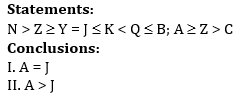(a) Both conclusions I and II are true
(b) Only conclusion II is true
(c) Neither conclusion I nor II is true
(d) Either conclusions I or II is true
(e) Only conclusions I is true

Q8.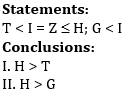(a) Both conclusions I and II are true
(b) Either conclusion I or II is true
(c) Neither conclusion I nor II is true
(d) Only conclusion I is true
(e) Only conclusion II is true

Q9.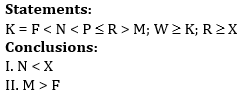(a) Only conclusion II is true
(b) Either conclusion I or II is true
(c) Both conclusions I and II are true
(d) Neither conclusion I nor II is true
(e) Only conclusion I is true

Q10.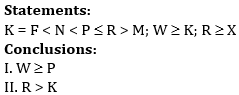(a) Neither conclusion I nor II is true
(b) Both conclusions I and II are true
(c) Only conclusion II is true
(d) Either conclusion I or II is true
(e) Only conclusion I is true

Solutions

Solutions (1-5):
Sol. In this input output question two numbers are arranged in each step following a certain pattern. Let us understand the logic behind it-
* Even numbers are arranged at the right end, from left to right in ascending order after adding one in each number (Numbers are picked from lowest to highest).
* Odd numbers are arranged at the left end, from right to left in ascending order after the digits are interchanged within the number (Numbers are picked from lowest to highest).

Input: 86 14 53 24 45 17 32 27 74 93
Step I: 71 86 53 24 45 32 27 74 93 15
Step II: 72 71 86 53 45 32 74 93 15 25
Step III: 54 72 71 86 53 74 93 15 25 33
Step IV: 35 54 72 71 86 93 15 25 33 75
Step V: 39 35 54 72 71 15 25 33 75 87

S1. Ans. (c)
S2. Ans. (b)
S3. Ans. (a)
S4. Ans. (c)
S5. Ans. (e)

Solutions (6-10):
S6. Ans. (c)
S7. Ans. (d)
S8. Ans. (a)
S9. Ans. (d)
S10. Ans. (c)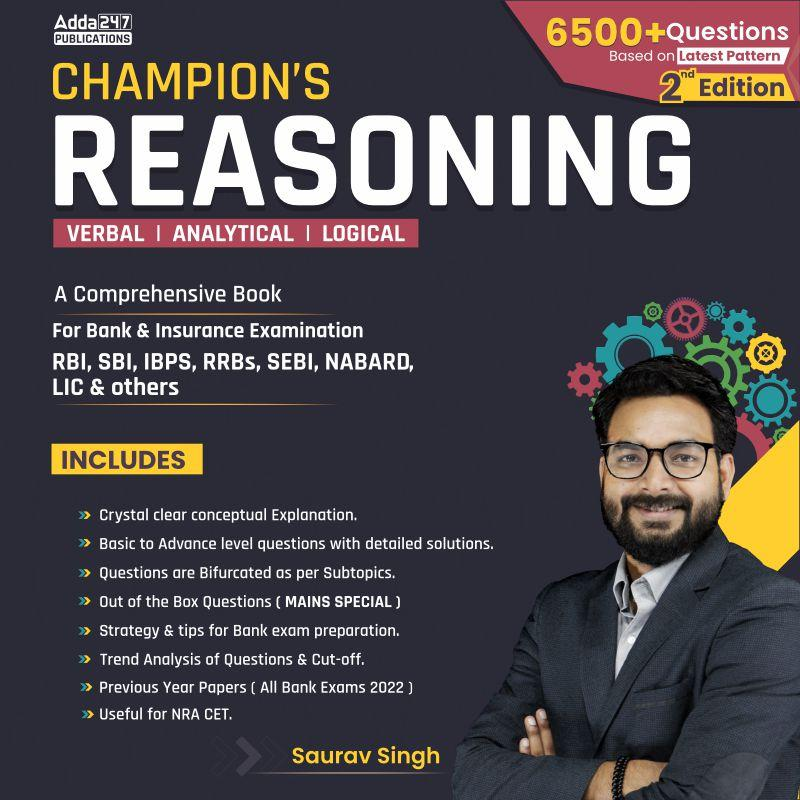## FAQs

### When will the IBPS PO prelims 2023 be conducted?

IBPS PO Prelims will be conducted on 23, 30 September, and 1 October 2023.

#### Congratulations!Union Budget 2023-24: Free PDF